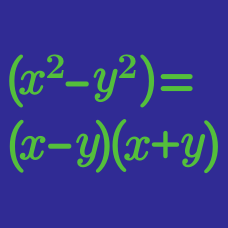Algebra

# Polynomial Factoring: Level 2 Challenges

$\large \sqrt a + b = 7 \\ \large \sqrt b + a = 11$

If $$a$$ and $$b$$ are real numbers that satisfy the equation above, what is the value of $$a$$ and $$b$$ respectively?

Given that $$6y^2=2014$$, find the value of

$\frac{9\big(y^4+6y^3+9y^2\big)}{y^2+6y+9}.$

$\large a^3 + b^3 = 2593080, \ \ a + b = 210, \ \ \ \ \ ab = \ ?$

Given that $$a+b=1$$ and $$a^2+b^2=2$$, what is the value of $$a^7+b^7$$?

If $$x^2 - 5x - 1 = 0$$, then find the value of $$x^2 + \frac{1}{x^2}.$$

×

Problem Loading...

Note Loading...

Set Loading...